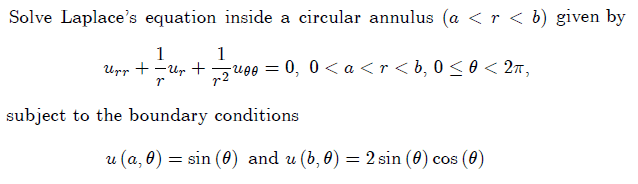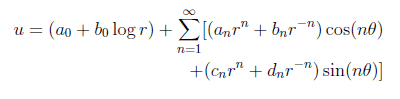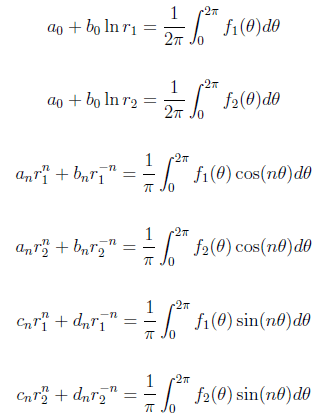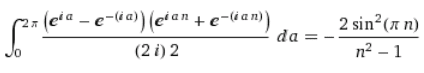# Laplace's equation inside a circular annulus

• temaire
In summary, the homework equations show that a sinusoidal function can be written as a sum of sin and cosine terms, with the cosine term being periodic in pi. However, the sinusoidal function is not periodic in pi.f

## Homework Statement## Homework Equations

General solution:Fourier series:where $r_{1}=a$, $r_{2}=b$, $f_{1}(\theta)=sin(\theta)$, and $f_{2}(\theta)=2sin(\theta)cos(\theta)$.

## The Attempt at a Solution

By evaluating the Fourier series shown above, I determined that $a_{o}=b_{o}=a_{n}=b_{n}=c_{n}=d_{n}=0$.

Is this correct?

Last edited:
Well, no. You could guess that for yourself because that would mean the answer is identically 0, which is not awfully likely for the diff eq is it?

I looked at ##\int_0^{2\pi}sin\theta cos{n \theta}d\theta ## . The best way to evaluate it is to express both the sin and cos in exponential form: ##sinx = \frac{e^x-e^{-x}}{2i}## and similarly for the cosnx. Multiply it all out and regroup your terms. You'll have a sin and a cosh. The former is periodic in ##\pi## the latter is not.

Do try again.

Well, no. You could guess that for yourself because that would mean the answer is identically 0, which is not awfully likely for the diff eq is it?

I looked at ##\int_0^{2\pi}sin\theta cos{n \theta}d\theta ## . The best way to evaluate it is to express both the sin and cos in exponential form: ##sinx = \frac{e^x-e^{-x}}{2i}## and similarly for the cosnx. Multiply it all out and regroup your terms. You'll have a sin and a cosh. The former is periodic in ##\pi## the latter is not.

Do try again.

I evaluated the integral you mentioned after expressing both the sin and cos in exponential form as follows:Isn't this still periodic in $\pi$?

How about ##2 \sin \theta \cos n \theta = \sin ((n+1)\theta) - \sin ((n-1) \theta)##?

How about ##2 \sin \theta \cos n \theta = \sin ((n+1)\theta) - \sin ((n-1) \theta)##?

Isn't that still always going to be zero at all n, where n is an integer and can't equal +-1, after integrating?

I evaluated the integral you mentioned after expressing both the sin and cos in exponential form as follows:Isn't this still periodic in $\pi$?

****
Here's what I have:

##sinx \cdot cos(nx)##= ##\frac{1}{4i}[(e^{ix}-e^{-ix}) \cdot (e^{inx}+e^{-inx})] ## = ##\frac{1}{4i}[(e^{i(n+1)x} - e^{-i(n+1)x}) + (e^{(n+1)x} - e^{-(n+1)x})]##

Integrating up the last expression and evaluating at 0 and ##2\pi ## gives

## \frac{1}{4(n+1)i}(e^{2(n+1)\pi} + e^{-2(n+1)\pi}) ##

This is not zero.

Try to be very careful when you do your integration.. Also, note that the product of two periodic functions is periodic, but not necessarily in the period of either.

Isn't that still always going to be zero at all n, where n is an integer and can't equal +-1, after integrating?
Quite so, so maybe something else is wrong. But it's much easier than writing out all those exponentials.

****
Here's what I have:

##sinx \cdot cos(nx)##= ##\frac{1}{4i}[(e^{ix}-e^{-ix}) \cdot (e^{inx}+e^{-inx})] ## = ##\frac{1}{4i}[(e^{i(n+1)x} - e^{-i(n+1)x}) + (e^{(n+1)x} - e^{-(n+1)x})]##
Not right. You should get some n-1 terms.

Not right. You should get some n-1 terms.

You are right, and I'm the one who should be careful about integrals. I do not know by what slip of the mind I lost the i's in the exponents. The other integral is 0 also.

So temaire is right, they are all 0, unless ##r_1 = r_2## (which isn't really an annulus,but whatever).